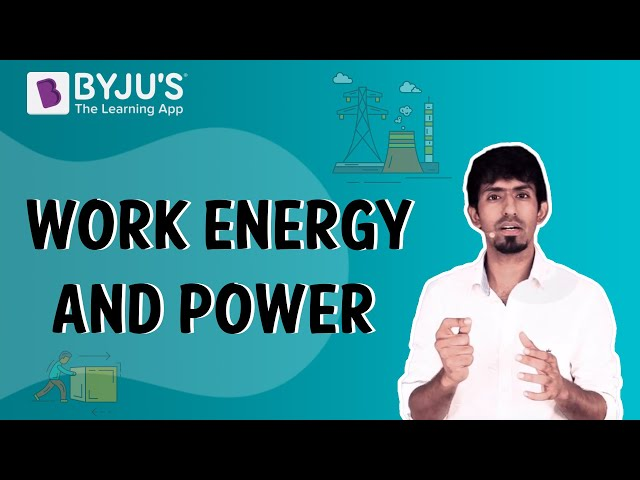# Difference Between Work and Energy

There is a significant difference between work and energy. Work is the transferring of an amount of energy with the help of a force covering a particular distance in a direction. Energy is also referred to as the force that works at a certain distance. Both of these can be termed scalar units. For work to occur, there must be a force and movement from one place to another.

## Difference Between Work and Energy

There are basically several types of energy such as potential, kinetic energy, nuclear energy, solar energy, electrical energy, etc. Kinetic energy is when an object is in motion and potential energy is when the object is at rest. On the other hand, there is only one type of work. Both these quantities are measured in joules.

 Difference Between Work and Energy Work Energy It is described as the action done on the object causing some displacement It is described as a property of a system or the ability to do work Work = force x distance There are various equations depending upon the types of energy The components of the force are parallel to the displacement Energy is the result of the work performed If the applied force is in the same direction as the displacement, then work is positive and if the applied force is in the opposite direction of the displacement then work is negative There is no direction component in energy.

These were some differences between Work and Energy. If you wish to find out more, download BYJU’S The Learning App.

RELATED ARTICLES:

## Know more about work, energy and power from the video.## Frequently Asked Questions – FAQs

Q1

### What is work?

Work is the transferring of an amount of energy with the help of a force covering a particular distance in a direction.
Q2

### What is the formula for work?

Work = force × distance
Q3

### What is power?

Power is the amount of energy transferred per unit of time.
Q4

### What is energy?

In classical mechanics, energy is a measurable property that is transmitted to a system or an object, expressed in the form of work.
Q5

### What are the two main types of energy?

Potential energy and kinetic energy are the two main types of energy.
Q6

### Explain work and energy in terms of their direction.

If the applied force is in the same direction as the displacement, then work is positive. If the applied force is in the opposite direction as the displacement, that work is negative. In the case of energy, there is no relevance to its direction.
Test your knowledge on Work and energy differences